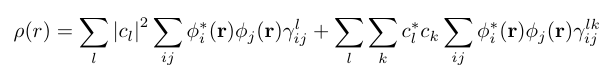# 1 particle reduced density matrices of EOM-CCSD states

Dear all,
I’m trying to plot the density of a superposition state/wave-packet (WP) formed by valence-excited EOM-CCSD states. I’ve done a short derivation which resulted in the equation shown in the attached picture. The coefficients c_k can be obtained from my theory and the cube files of the MO (i.e. \phi) easily from Qchem. What I need is to print out the 1-particle reduced density matrix (1RDM i.e. \gamma^l_{ij}-left term) and the 1-particle transition density matrix (1TDM i.e. \gamma^{lk}_{ij}-right term). Both the 1RDM and 1TDM are relative to the single valence-excited EOM-CCSD states. Is it possible to make Qchem print these matrices out?
Thank you very much. Have a nice day.All of these matrices are computed by Q-Chem when evaluating state and transition properties with EOM, however I do not think there’s a user-facing way to obtain them.

Don’t we have functionality to print cube files for EOM DMs? I recall we used to do that routinely. In any case, this should be very easy to accomplish by adding print statements.

If you activate state_analysis=true and gui=2, then I think the DMs are written to the fchk file.

To get all the DMs, you’d also have to activate cc_eom_prop and cc_state_to_state_prop

-Felix